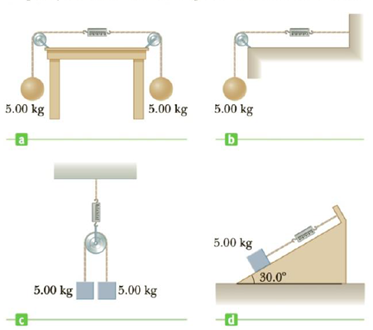Chapter 4, Problem 58P

Chapter
Section
Textbook Problem

The systems shown in Figure P4.58 are in equilibrium. If the spring scales are calibrated in newtons, what do they read? Ignore the masses of the pulleys and strings and assume the pulleys and the incline in Figure P4.58d are frictionless.Figure P4.58

(a)

To determine
The reading on the spring scale.

Explanation

Given Info: Masses attached are 5 kg.

The free body diagram is given below.

From the diagram, the tension (T) is,

T=mg

• m is the mass of the block.
• g is the acceleration due to gravity.

Substitute 5 kg for m and 9.8ms2 in the above expression to get T

(b)

To determine
The reading on the spring scale.

(c)

To determine
The reading on the spring scale.

(d)

To determine
The reading on the spring scale.

Still sussing out bartleby?

Check out a sample textbook solution.

See a sample solution

The Solution to Your Study Problems

Bartleby provides explanations to thousands of textbook problems written by our experts, many with advanced degrees!

Get Started

Cod is one of the richest sources of the beneficial fatty acids, EPA and DHA. T F

Nutrition: Concepts and Controversies - Standalone book (MindTap Course List)

___ is the actual path length. (2.2)

An Introduction to Physical Science

What is a consensus sequence in transcription?

Introduction to General, Organic and Biochemistry

Why can’t a white dwarf have a mass greater than 1.4 solar masses?

Horizons: Exploring the Universe (MindTap Course List)